Скачать презентацию Thomson Parthood and Identity Across Time Thomson s aim

7036457daac87d3ee4408c848de4991c.ppt

• Количество слайдов: 10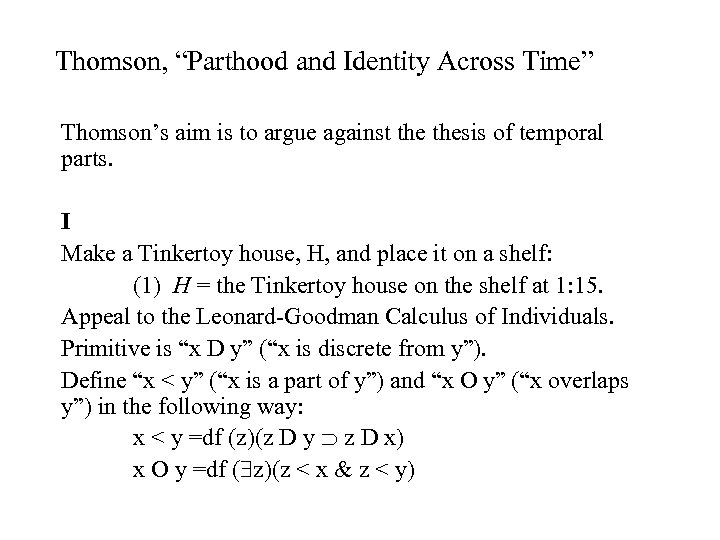Thomson, “Parthood and Identity Across Time” Thomson’s aim is to argue against thesis of temporal parts. I Make a Tinkertoy house, H, and place it on a shelf: (1) H = the Tinkertoy house on the shelf at 1: 15. Appeal to the Leonard-Goodman Calculus of Individuals. Primitive is “x D y” (“x is discrete from y”). Define “x < y” (“x is a part of y”) and “x O y” (“x overlaps y”) in the following way: x < y =df (z)(z D y z D x) x O y =df ( z)(z < x & z < y)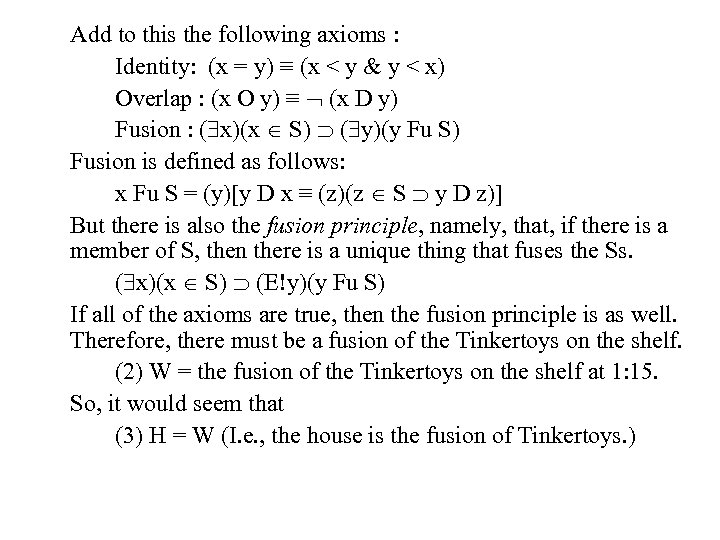Add to this the following axioms : Identity: (x = y) ≡ (x < y & y < x) Overlap : (x O y) ≡ (x D y) Fusion : ( x)(x S) ( y)(y Fu S) Fusion is defined as follows: x Fu S = (y)[y D x ≡ (z)(z S y D z)] But there is also the fusion principle, namely, that, if there is a member of S, then there is a unique thing that fuses the Ss. ( x)(x S) (E!y)(y Fu S) If all of the axioms are true, then the fusion principle is as well. Therefore, there must be a fusion of the Tinkertoys on the shelf. (2) W = the fusion of the Tinkertoys on the shelf at 1: 15. So, it would seem that (3) H = W (I. e. , the house is the fusion of Tinkertoys. )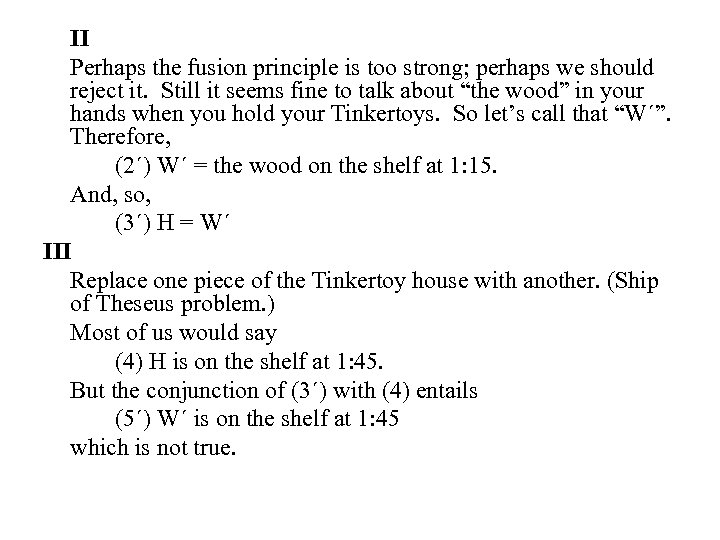II Perhaps the fusion principle is too strong; perhaps we should reject it. Still it seems fine to talk about “the wood” in your hands when you hold your Tinkertoys. So let’s call that “W´”. Therefore, (2´) W´ = the wood on the shelf at 1: 15. And, so, (3´) H = W´ III Replace one piece of the Tinkertoy house with another. (Ship of Theseus problem. ) Most of us would say (4) H is on the shelf at 1: 45. But the conjunction of (3´) with (4) entails (5´) W´ is on the shelf at 1: 45 which is not true.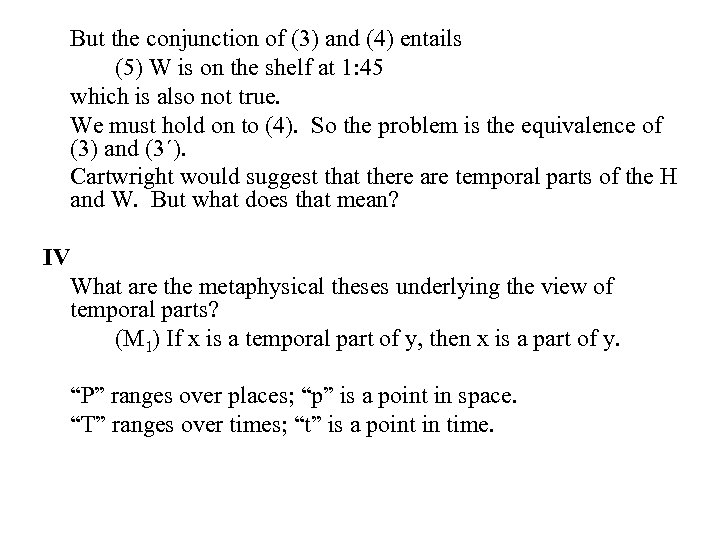But the conjunction of (3) and (4) entails (5) W is on the shelf at 1: 45 which is also not true. We must hold on to (4). So the problem is the equivalence of (3) and (3´). Cartwright would suggest that there are temporal parts of the H and W. But what does that mean? IV What are the metaphysical theses underlying the view of temporal parts? (M 1) If x is a temporal part of y, then x is a part of y. “P” ranges over places; “p” is a point in space. “T” ranges over times; “t” is a point in time.A definition of cross-sectional temporal part leads to the second metaphysical thesis. (M 2) (T)[y exists through T ( x)(x exists through T & no part of x exists outside T & (t)(t is in T (P)(y exactly occupies P at t x exactly occupies P at t))] Now we need to ensure uniqueness. (M 3) If x is a part of y and y is a part of x, then x is identical with y.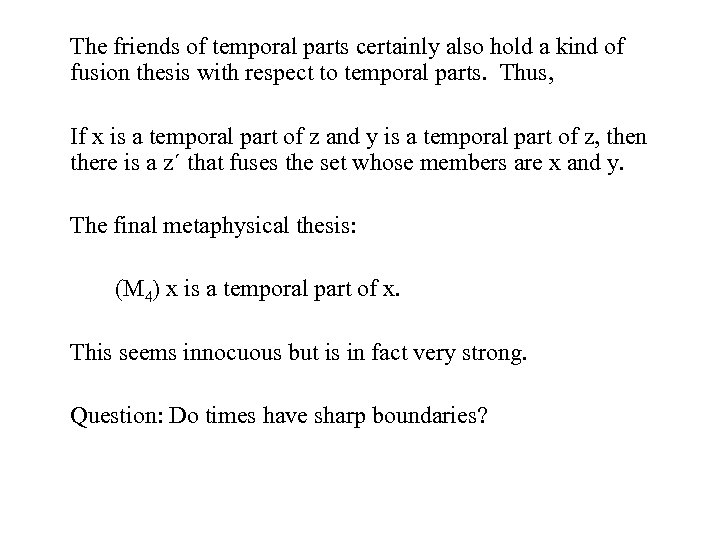The friends of temporal parts certainly also hold a kind of fusion thesis with respect to temporal parts. Thus, If x is a temporal part of z and y is a temporal part of z, then there is a z´ that fuses the set whose members are x and y. The final metaphysical thesis: (M 4) x is a temporal part of x. This seems innocuous but is in fact very strong. Question: Do times have sharp boundaries?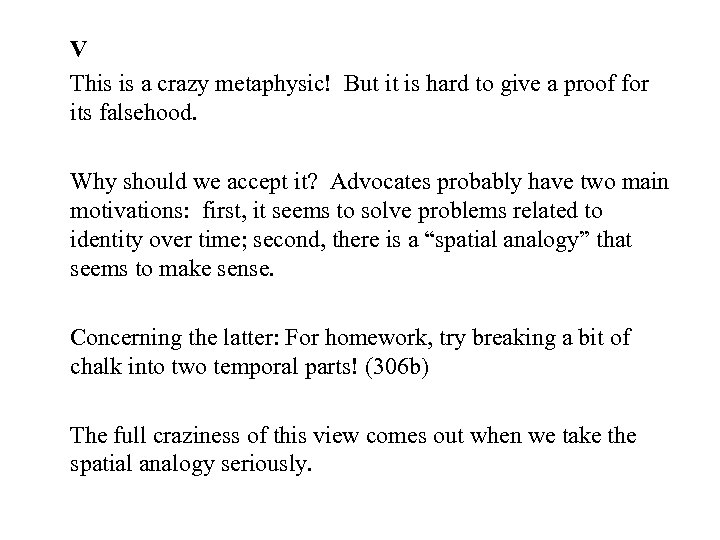V This is a crazy metaphysic! But it is hard to give a proof for its falsehood. Why should we accept it? Advocates probably have two main motivations: first, it seems to solve problems related to identity over time; second, there is a “spatial analogy” that seems to make sense. Concerning the latter: For homework, try breaking a bit of chalk into two temporal parts! (306 b) The full craziness of this view comes out when we take the spatial analogy seriously.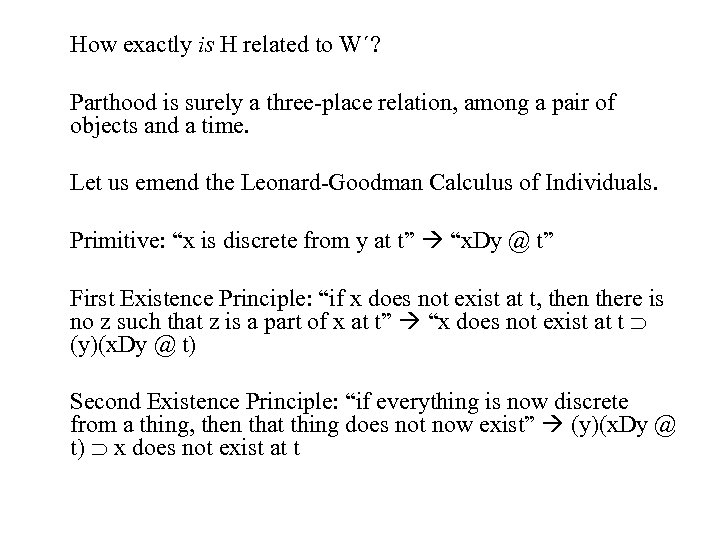How exactly is H related to W´? Parthood is surely a three-place relation, among a pair of objects and a time. Let us emend the Leonard-Goodman Calculus of Individuals. Primitive: “x is discrete from y at t” “x. Dy @ t” First Existence Principle: “if x does not exist at t, then there is no z such that z is a part of x at t” “x does not exist at t (y)(x. Dy @ t) Second Existence Principle: “if everything is now discrete from a thing, then that thing does not now exist” (y)(x. Dy @ t) x does not exist at t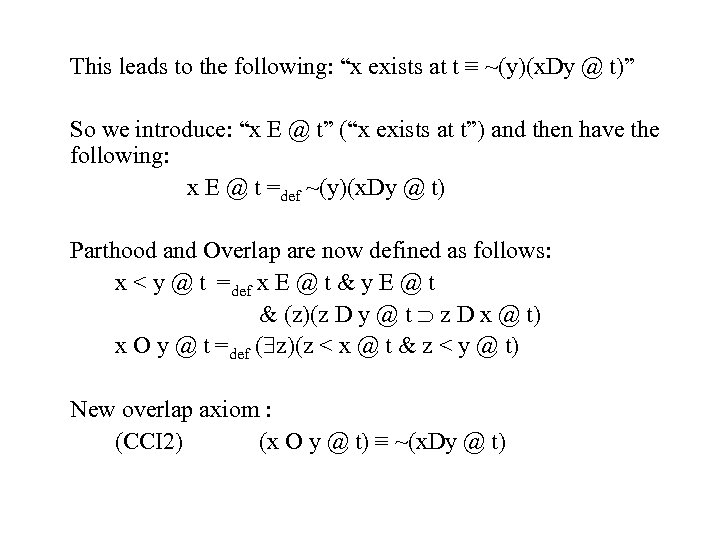This leads to the following: “x exists at t ≡ ~(y)(x. Dy @ t)” So we introduce: “x E @ t” (“x exists at t”) and then have the following: x E @ t =def ~(y)(x. Dy @ t) Parthood and Overlap are now defined as follows: x < y @ t =def x E @ t & y E @ t & (z)(z D y @ t z D x @ t) x O y @ t =def ( z)(z < x @ t & z < y @ t) New overlap axiom : (CCI 2) (x O y @ t) ≡ ~(x. Dy @ t)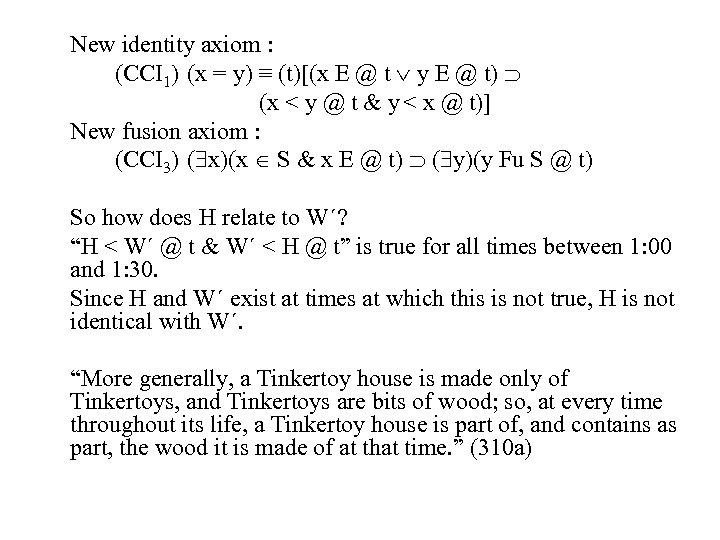New identity axiom : (CCI 1) (x = y) ≡ (t)[(x E @ t y E @ t) (x < y @ t & y < x @ t)] New fusion axiom : (CCI 3) ( x)(x S & x E @ t) ( y)(y Fu S @ t) So how does H relate to W´? “H < W´ @ t & W´ < H @ t” is true for all times between 1: 00 and 1: 30. Since H and W´ exist at times at which this is not true, H is not identical with W´. “More generally, a Tinkertoy house is made only of Tinkertoys, and Tinkertoys are bits of wood; so, at every time throughout its life, a Tinkertoy house is part of, and contains as part, the wood it is made of at that time. ” (310 a)